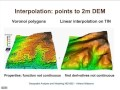Interpolation methods lecture (Video) - Monde Geospatial

Trending

# Interpolation methods lecture (Video)

In the mathematical field of numerical analysis, interpolation is a method of constructing new data points within the range of a discrete set of known data points.

In engineering and science, one often has a number of data points, obtained by sampling or experimentation, which represent the values of a function for a limited number of values of the independent variable. It is often required to interpolate (i.e., estimate) the value of that function for an intermediate value of the independent variable.

A different problem which is closely related to interpolation is the approximation of a complicated function by a simple function. Suppose the formula for some given function is known, but too complex to evaluate efficiently. A few known data points from the original function can be used to create an interpolation based on a simpler function. When a simple function is used to estimate data points from the original, interpolation errors are usually present; however, depending on the problem domain and the interpolation method used, the gain in simplicity may be of greater value than the resultant loss in precision

Monde GEOspatial 2018
Interpolation methods lecture (Video)Reviewed by world on February 18, 2018 Rating: 5 In the mathematical field of numerical analysis, interpolation is a method of constructing new data points within the range of a discrete s...